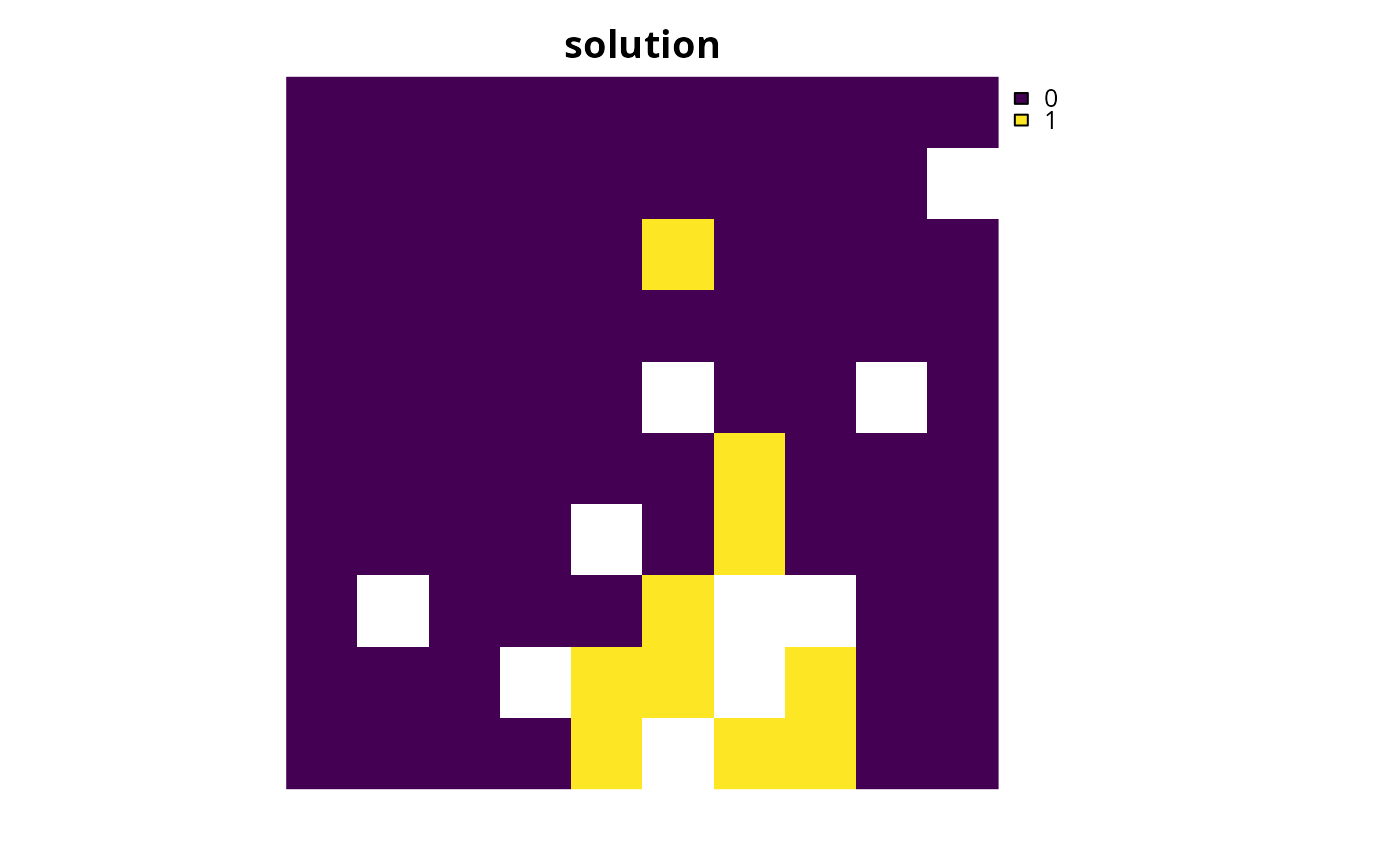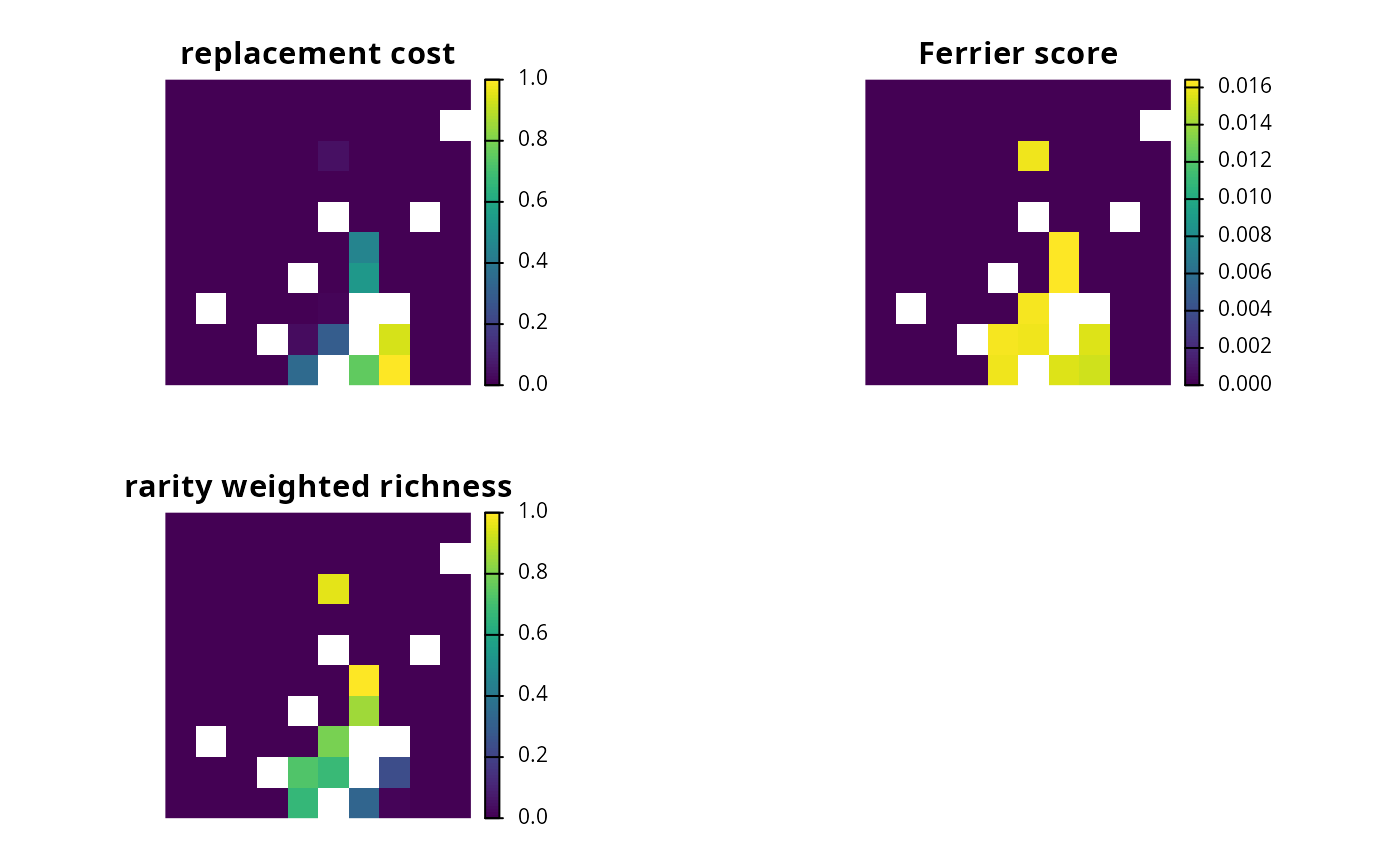Importance scores (also known as irreplaceability scores) can be used to assess the relative importance of planning units selected in a solution to a conservation planning problem().

## Details

The following methods are available for calculating importance scores:

eval_replacement_importance()

Calculate importance scores using replacement costs (based on Cabeza and Moilanen 2006). These scores quantify the change in the objective function (e.g., additional costs required to meet feature targets) of the optimal solution if a given planning unit in a solution cannot be acquired. They can (i) account for the cost of different planning units, (ii) account for multiple management zones, (iii) apply to any objective function, and (iv) identify truly irreplaceable planning units (denoted with infinite values).

eval_ferrier_importance()

Calculate importance scores following Ferrier et al. (2000). These scores measure importance based on how critical planning units are for meeting targets. They can only be applied to conservation problems with a minimum set objective and a single zone (i.e., the classic Marxan-type problem). Furthermore---unlike the replacement cost scores---these scores provide a score for each feature within each planning unit, providing insight into why certain planning units are more important than other planning units.

eval_rare_richness_importance()

Calculate importance scores using the rarity weighted richness metric (based on Williams et al. 1996). These score are simply a measure of biodiversity. They do not account for planning costs, multiple management zones, objective functions, or feature targets (or weightings). They merely describe the spatial patterns of biodiversity, and do not account for many of the factors needed to quantify the importance of a planning unit for achieving conservation goals.

Broadly speaking, we recommend using replacement cost scores where possible. This is because they can be applied to any type of conservation planning problem -- regardless of the objective function or number of zones considered in the problem -- and measure planning unit importance based on degradation of the prioritization. Although the replacement cost scores can be calculated for small and moderate sized problems (e.g., less than 30,000 planning units), they may not be feasible for particularly large problems (e.g., more than 100,000 planning units). In such cases, we recommend calculating importance scores using the Ferrier method. This is because the Ferrier method can be calculated relatively quickly for large-sized problems and it explicitly accounts for representation targets. We only recommend using the rarity weighted richness metric when neither of the other two methods can be used.

Other overviews: constraints, decisions, objectives, penalties, portfolios, solvers, summaries, targets

## Examples

# \dontrun{
data(sim_pu_raster, sim_pu_polygons, sim_features)

# build minimal conservation problem with raster data
p1 <- problem(sim_pu_raster, sim_features) %>%
add_default_solver(gap = 0, verbose = FALSE)

# solve the problem
s1 <- solve(p1)

# plot solution
plot(s1, main = "solution", axes = FALSE, box = FALSE)# calculate importance scores using replacement cost scores
ir1 <- eval_replacement_importance(p1, s1)

# calculate importance scores using Ferrier et al 2000 method,
# and extract the total importance scores
ir2 <- eval_ferrier_importance(p1, s1)[["total"]]

# calculate importance scores using rarity weighted richness scores
ir3 <- eval_rare_richness_importance(p1, s1)

# plot importance scores
plot(stack(ir1, ir2, ir3), axes = FALSE, box = FALSE,
main = c("replacement cost", "Ferrier score",
"rarity weighted richness"))# }